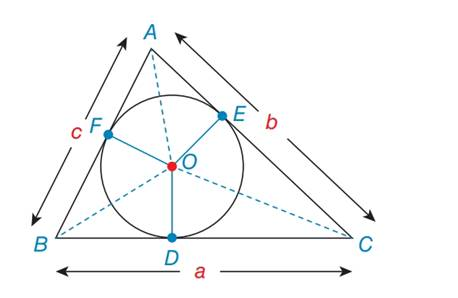Chapter 7.2, Problem 44EElementary Geometry For College St...

7th Edition
Alexander + 2 others
ISBN: 9781337614085

Solutions

Chapter
SectionElementary Geometry For College St...

7th Edition
Alexander + 2 others
ISBN: 9781337614085
Textbook Problem

For exercises 44 to 46, O is the inscribed circle for ∆ A B C . In exercises 45 and 46, use the result from 44.Explain why ∆ A O F ≅ A O E .To determine

To explain:

Why ΔAOEΔAOF

Explanation

Definition:

Angle Angle Side (AAS):

If two angles and a non included side of a triangle are congruent to two angles and the corresponding non included side of another triangle, then the two triangles are congruent by angle angle side postulate.

Given:

Explain ΔAOEΔAOF

Calculation:

Consider the triangle ΔAOEandΔAOF

Still sussing out bartleby?

Check out a sample textbook solution.

See a sample solution

The Solution to Your Study Problems

Bartleby provides explanations to thousands of textbook problems written by our experts, many with advanced degrees!

Get Started

Find more solutions based on key concepts